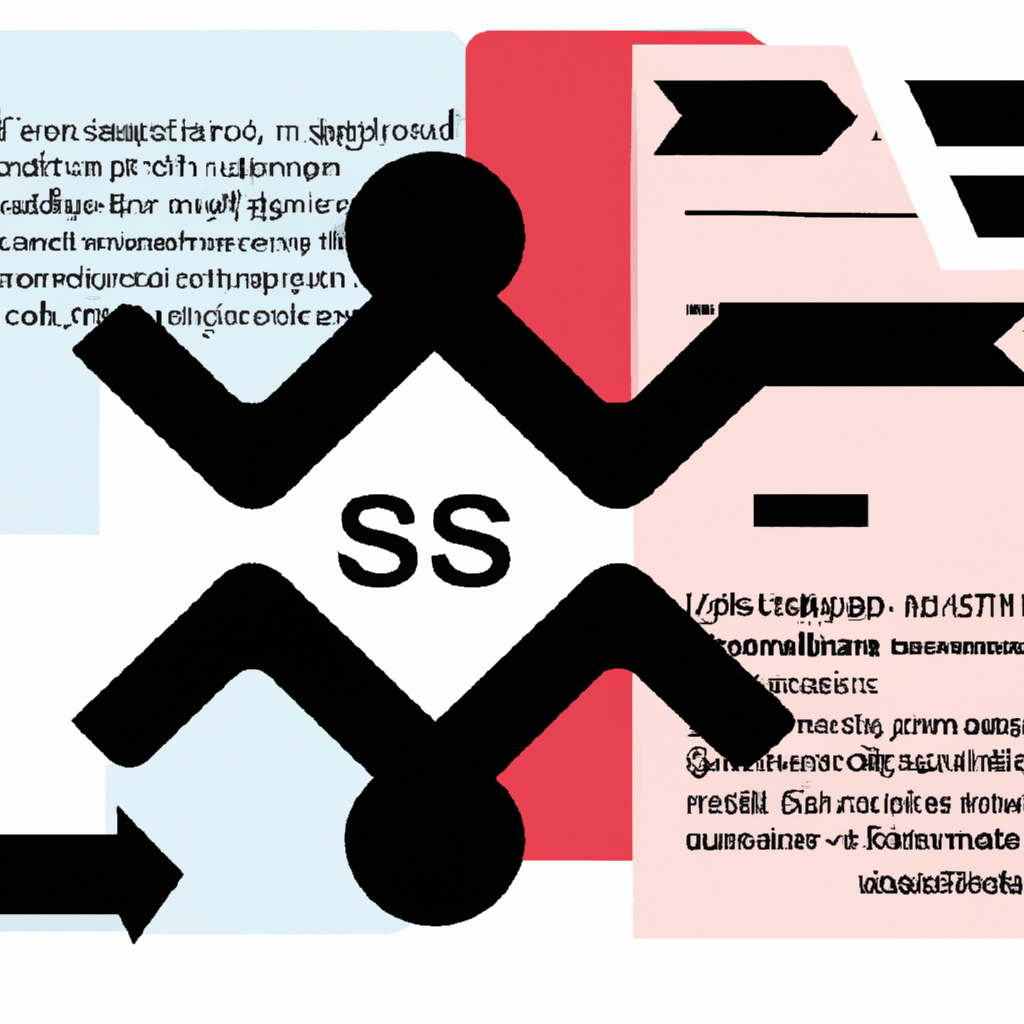Python

# Understanding Python’s list().sort() Method: A Comprehensive Guide

In this tutorial, we will delve into the usage, functionality, and proper implementation of Python’s built-in method for sorting lists – `list().sort()`. This powerful tool is essential for any Python programmer looking to organize data in a meaningful way.

## What is list().sort()?

The `list().sort()` method in Python is used to sort the elements present within a list in either ascending order (which is default) or descending order. It modifies the original list and does not return any value.

### Syntax

``````
list.sort([key=...][, reverse=...])
```
```

This method accepts two parameters:

• Key: A function that serves as a key or basis of sort comparison. It’s optional.
• Reverse: If set true, then the sorted list elements are reversed (or sorted in descending order). It’s also optional.

## A Basic Example of list().sort()

``````
numbers = [4, 2, 9, 7]
numbers.sort()
print(numbers)
# Output: [2, 4, 7, 9]
```
```

In this example above, we have a simple unsorted list called ‘numbers’. We apply the `.sort()` method directly on it without passing any parameters. The result is an ordered (ascending) version of our original list.

## List Sorting with Key Parameter

You can pass a function as a key parameter which will be used for sorting purposes. Let’s see an example:

``````
words = ['apple', 'banana', 'cherry', 'date']
words.sort(key=len)
print(words)
# Output: ['date', 'apple', 'cherry', 'banana']
```
```

In this case, we’re sorting a list of words based on their length by passing the built-in function `len()` as the key parameter.

## List Sorting in Descending Order

If you want to sort your list in descending order, you can set the reverse parameter to True. Here’s how:

``````
numbers = [4, 2, 9, 7]
numbers.sort(reverse=True)
print(numbers)
# Output: [9, 7, 4, 2]
```
```

This time around our numbers are sorted in descending order.

### Conclusion

The `list().sort()` method is a versatile and powerful tool for organizing your data within Python. Whether you’re working with numerical data or strings, understanding how to properly use this method will greatly enhance your ability to manipulate and analyze data effectively.